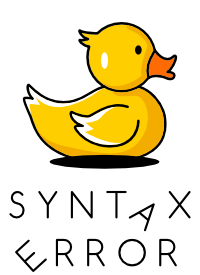Juha-Matti Santala

# True, True, True == (True, True, True) in PythonA friend shared this interesting piece of Python code in our Telegram chat today:

``````>>> True, True, True == (True, True, True)
(True, True, False)``````

(If you want to figure it out yourself first, stop reading now and return once you want to learn or confirm your thoughts.)

So what's happening here? Let's first take a look at what are tuples in Python.

Tuples are immutable sequences, typically used to store collections of heterogeneous data (such as the 2-tuples produced by the `enumerate()` built-in). Tuples are also used for cases where an immutable sequence of homogeneous data is needed (such as allowing storage in a `set` or `dict` instance).

There are a couple of ways to create a tuple, two of which are seemingly used in this little brainteaser:

``````>>> True, True, True # Using commas
(True, True, True)
>>> (True, True, True) # Using parenthesis
(True, True, True)
>>> tuple(True, True, True) # Using tuple constructor``````

So when looking at the original piece of code, it seems that we're creating two tuples with three `True` in each and then comparing these items to each other. When comparing tuples, comparison is done item-by-item: first you compare items in index 0, then in index 1 and so on.

In reality, comparing two tuples however doesn't return a new tuple with the results of individual comparisons but a single boolean value `True` or `False`.

So what's actually happening when this code gets executed? To create a tuple, Python will evaluate each of its items and then store that data in a tuple.

``````# First Python sees there's a True
# Then there's another True with comma in between
# Then there's expression True == (True, True, True) which gets evaluated into False
# A tuple is created with values (True, True, False)
>>> True, True, True == (True, True, True)
(True, True, False)
``````

The comparison function, the use of tuples and the use of booleans is what makes this initially weird looking. If we take a look at an example of tuple creation with other values and operators, it becomes clearer.

``````>>> 1, 2, 5 + 7
(1, 2, 12)
``````

What happens here is exactly the same: each item is evaluated one by one, left to right and then stored as a tuple.

Thanks to Helio Loureiro for sharing this with me.Sign up for Syntax Error, a monthly newsletter that helps developers turn a stressful debugging situation into a joyful exploration.